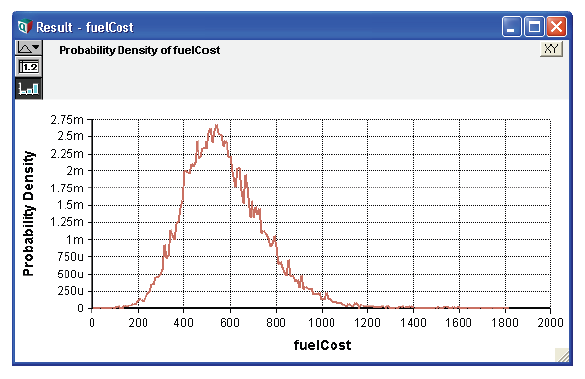# Sensitivity analysis functions

Sensitivity analysis enables you to examine the effect of a change in the value of an input variable on the values of its output variables. They do not require their parameters to be uncertain.

Examples: The examples in this section refer to the following variables:

gasPrice  Cost of gasoline per gallon within market fluctuations The average number of miles driven per year Fuel consumption averaged over driving conditions Annual cost of fuel

The probability density of fuelCost is shown below.## Dydx(y, x)

Returns the derivative of expression «y» with respect to variable «x», evaluated at mid values. This function returns the ratio of the change in y to a small change in «x» that affects «y». The “small change” is x/10000, or 1.0E-6 if x = 0. See Dydx.

Library: Special

Examples: Because fuelCost depends on mpg, a small change in mpg seems to have a modest negative effect on fuelCost:

Dydx(fuelCost, mpg) → -19.7

The reverse is not true, because mpg is not dependent on fuelCost. That is, fuelCost does not cause any change in mpg:

Dydx(Mpg, Fuelcost) → 0

In this model of fuelCost, a small change in gasPrice has by far the largest effect of all its inputs:

Dydx(fuelCost, gasPrice) → 428.6
Dydx(fuelCost, mpy) → 0.04643
Tip

When you evaluate Dydx in mid mode, the mid value for «x» is varied and the mid value of «y» is evaluated. In prob mode, the sample of «x» is varied and the sample for «y» is computed in prob mode. Therefore, when «y» is a statistical function of «x», care must be taken to ensure that the evaluation modes for «x» and «y» correspond. So, for example:

Y := DyDx(Kurtosis(Normal(0, X)), X)

would not produce the expected result. In this case, when evaluating «y» in determ mode, Kurtosis evaluates its parameter, and thus «x», in prob mode, resulting in a mis-match in computation modes. To get the desired result, you should explicitly use the mid value of «x»:

Y := DyDx(Kurtosis(Normal(0, Mid(X))), X)

## Elasticity(y, x)

Returns the percent change in variable «y» caused by a 1 percent change in a dependent variable «x». Mathematically, writing y(x) to emphasize that «y» is a function of «x», elasticity is defined as:

$\displaystyle{ Elasticity(y,x)=\lim_{u \to 0} \frac{1}{u} (\frac{y(x(1+u))-y(x)}{y(x)}) }$

When «x» is a positive scalar, but not when «x» is array-valued, Elasticity is related to Dydx in the following manner:

Elasticity(y, x) = Dydx(y, x)*(x/y)

Library: Special

Examples:

Elasticity(fuelCost, mpg) → -1
Elasticity(fuelCost, gasPrice) → 1

A 1% change in variables mpg and gasPrice cause about the same degree of change in fuelCost, although in opposite directions.

mpg is inversely proportional to the value of fuelCost, while gasPrice is proportional to it.

Tip
When you evaluate Elasticity in determ (mid) mode, the mid value for «x» is varied and the mid value of «y» is evaluated. In prob mode, the sample of «x» is varied and the sample for «y» is computed in prob mode. Therefore, when «y» is a statistical function of «x», care must be taken to ensure that the evaluation modes for «x» and «y» correspond.

## Whatif(e, v, vNew)

Returns the value of expression «e» when variable «v» is set to the value of «vNew». «v» must be a variable. It lets you explore the effect of a change to a value without changing it permanently. It restores the original definition of «v» after evaluating Whatif expression, so that there is no permanent change (and so causes no side effects).

Library: Special

Example:

Fuelcost → 557.1
Whatif(Fuelcost, Mpy, 14K) → 650

## WhatIfAll(e, vList, vNew)

Like Whatif, but it lets you examine a set of changes to a list of variables, «vList». It returns the mid value of «e» when each of variables in «vList» is assigned the value in «vNew» one at a time, with the remaining variables remaining at their nominal values. The result is indexed by «vList». If «vNew» is indexed by «vList», it assigns the corresponding value of «vNew» to each variable, letting you assign a different value to each variable in «vList». WhatIfAll is useful for performing ceteris paribus style sensitivity analysis, which varies one variable at a time, leaving the others at their initial value, such as in Tornado charts (see next section for an example).

Suppose Z is a function of A, B, and C, and we wish to examine the effect on Z when each input is varied, one at a time, by 10% from its nominal value. Define:

Variable Z := 10*A + B^2 + 5*C
Index L := [90%, 110%]
Variable V := [A, B, C]
MyTornado := WhatIfAll(Z, V, L*V)

Library: Special